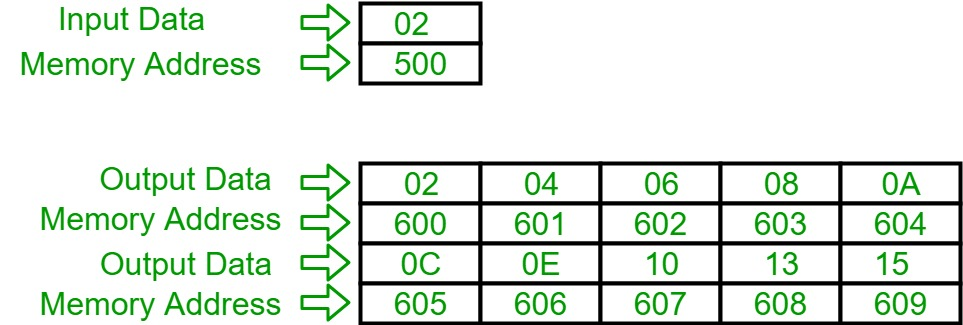Open in App
Not now

# 8086 program to print the table of input integer

• Difficulty Level : Easy
• Last Updated : 29 Oct, 2021

Problem – Write an assembly language program in 8086 to print the table of input integer.

Assumption – Suppose the inputted number is at memory location 500 and the table will be printed from starting location 600 till 609 in hexadecimal.

Example –Algorithm –

2. Store 00 in CH register.

3. Increment value of CH by 1 and move the content of [SI] into AH register.

4. Multiply content of AL and CH and store it in AX and then move content of AL into [DI], then increment value of DI by 1.

5. Compare the value of CH and 0A, if not equal then go to step number 3 otherwise halt the program.

Program –

Explanation –

1. MOV SI, 500: load 500 in SI.

2. MOV DI, 600: load 600 in DI.

3. MOV CH, 00: load 00 data in CH register.

4. INC CH: increment the value inside CH register by 1.

5. MOV AL, SI: move the content of SI into AL register.

6. MUL CH: multiply the contents of AL and CH register and store in AX register.

7. MOV [DI], AL: move the contents of AL register into [DI].

8. INC DI: increment the value of DI by 1.

9. CMP CH, 0A: subtract data inside CH register and 0A.